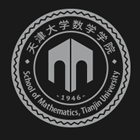﻿Complex Monge-Ampere equation and its geometric applications-天津大学数学学院

# Complex Monge-Ampere equation and its geometric applications

2019-08-05 10:42

In this series of lectures, we will introduce the complex Monge-Ampere equation on compact Kahler manifolds. Then we shall discuss geometric applications such as the Calabi-Yau theorem, the regularity of geodesics in the space of Kahler metrics and so on.### 联系我们